## 什么是数据流分析

• 在编译器中，通过数据流分析，编译器可以了解变量间的定义和使用关系，从而在编译期间提前计算部分常量传播 (Constant Propagation) 优化，降低程序运行时所需的计算量。
• 数据流分析也可以协助编译器检查某段代码是否可达 (在动态运行时能不能执行到)，编译器可以结合分析结果，移除部分不可达的代码
• 在软件开发和测试中，数据流分析作为静态分析方法，在程序不运行的情况下，对变量的声明、赋值、使用进行分析，并形成合理代码的改进建议

## 什么是基本块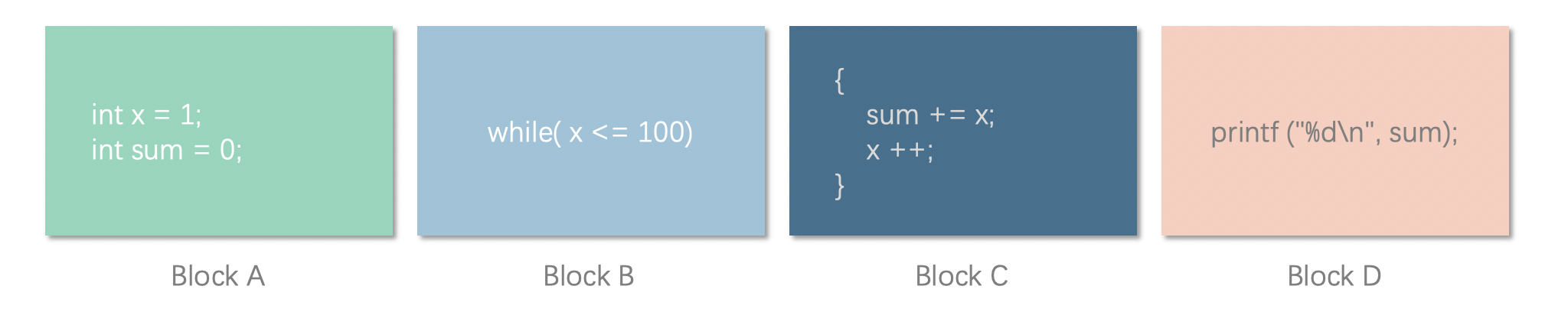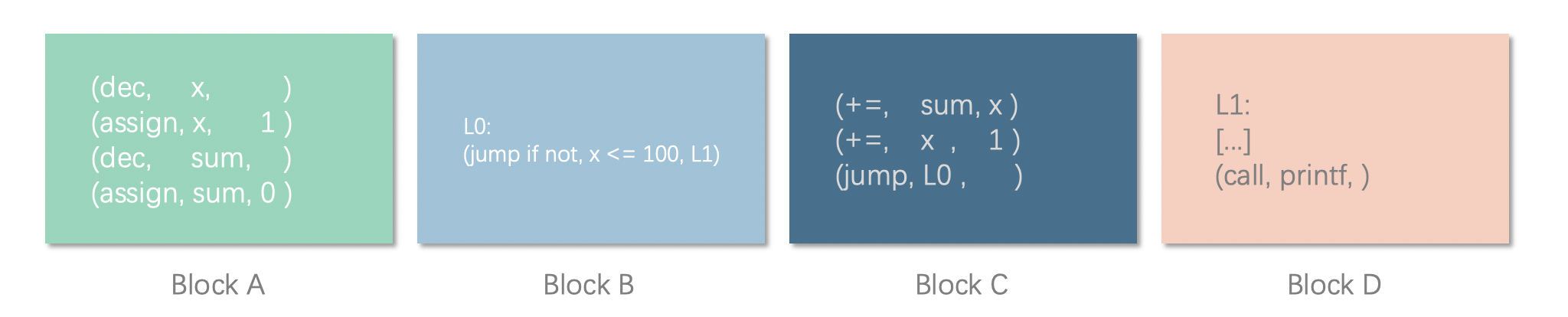## 从基本块到控制流图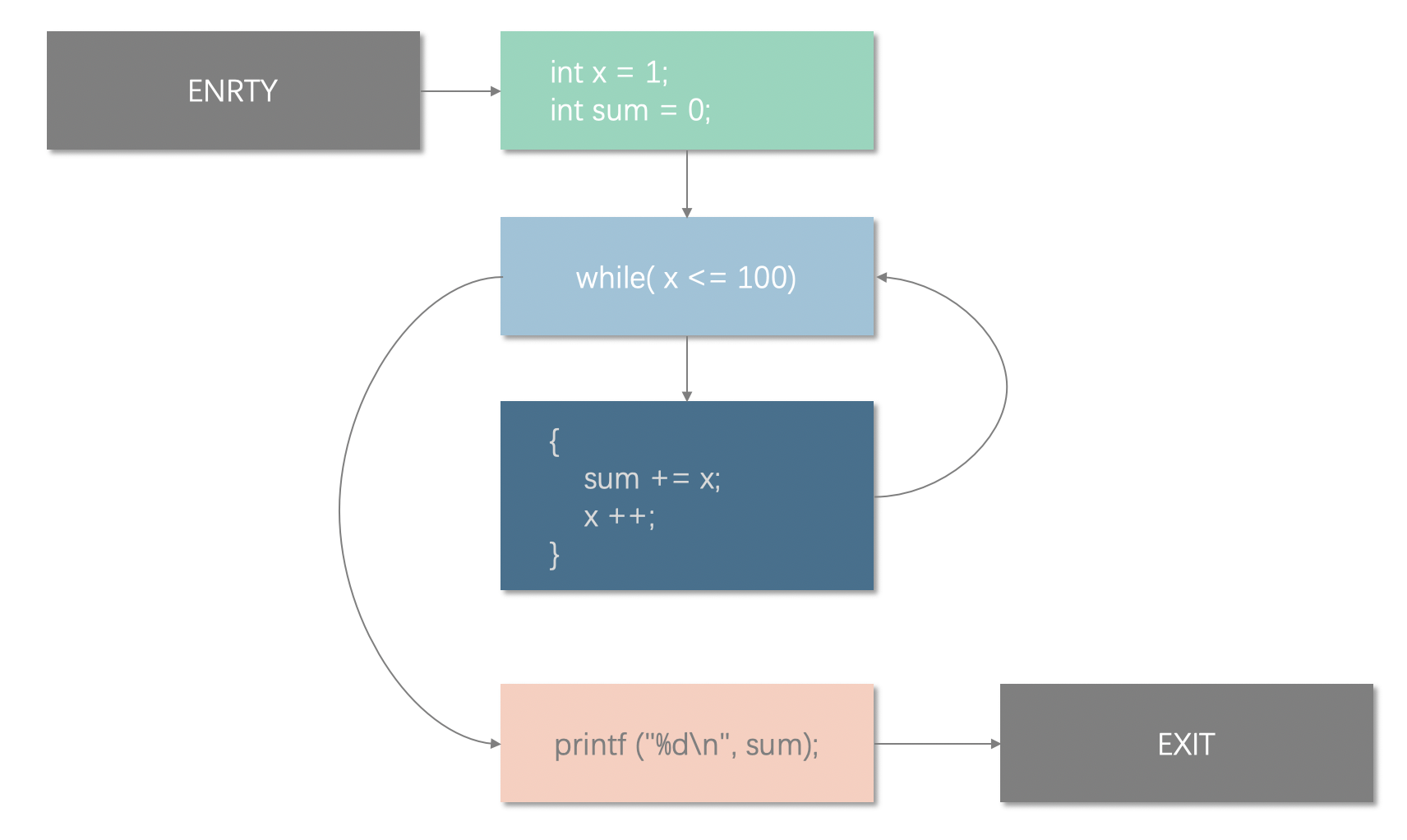## 定值？？使用？？？

• 定值 (definition, def): 一般指变量的赋值，一般包括变量声明和初始化、复制、形式参数列表等
• 使用 (use): 使用变量的值进行运算，一般包括表达式、逻辑判断条件，把变量作为参数传递，返回值等

$$def = { a, b} \ use = { a }$$

## 变量的 “生成” 和 “被杀死”

• 生成 (Genenrate, gen) - 定值的生成，也就是变量的定义、初始化
• 杀死 (Kill) - 路径上的前驱节点以及给出的定值在本语句中被改写，即前驱节点生成的定值被 “杀” 掉了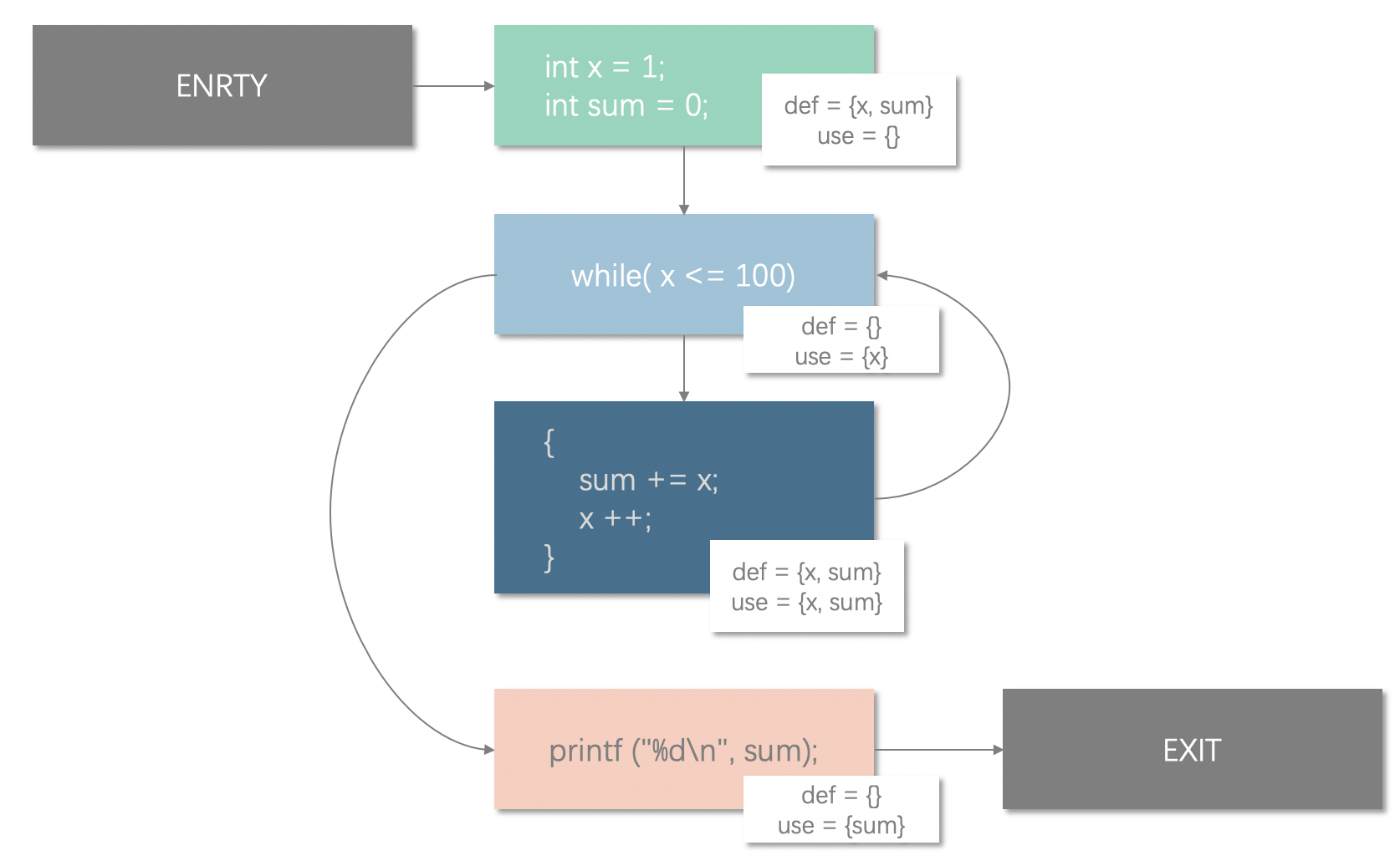$$Gen[A] = {x, sum}$$

$$Kill[C] = {x, sum}$$

## 数据流分析的方式

• $IN[B_{i}]$ 为路径上前驱节点 $B_i$ 发出的到达当前节点的定值集合
• $OUT[B_{j}]$ 为当前节点向路径上的后继节点 $B_j$ 发出的最新定值集合

$$\bigcup_{i}^{0..n}IN[i]$$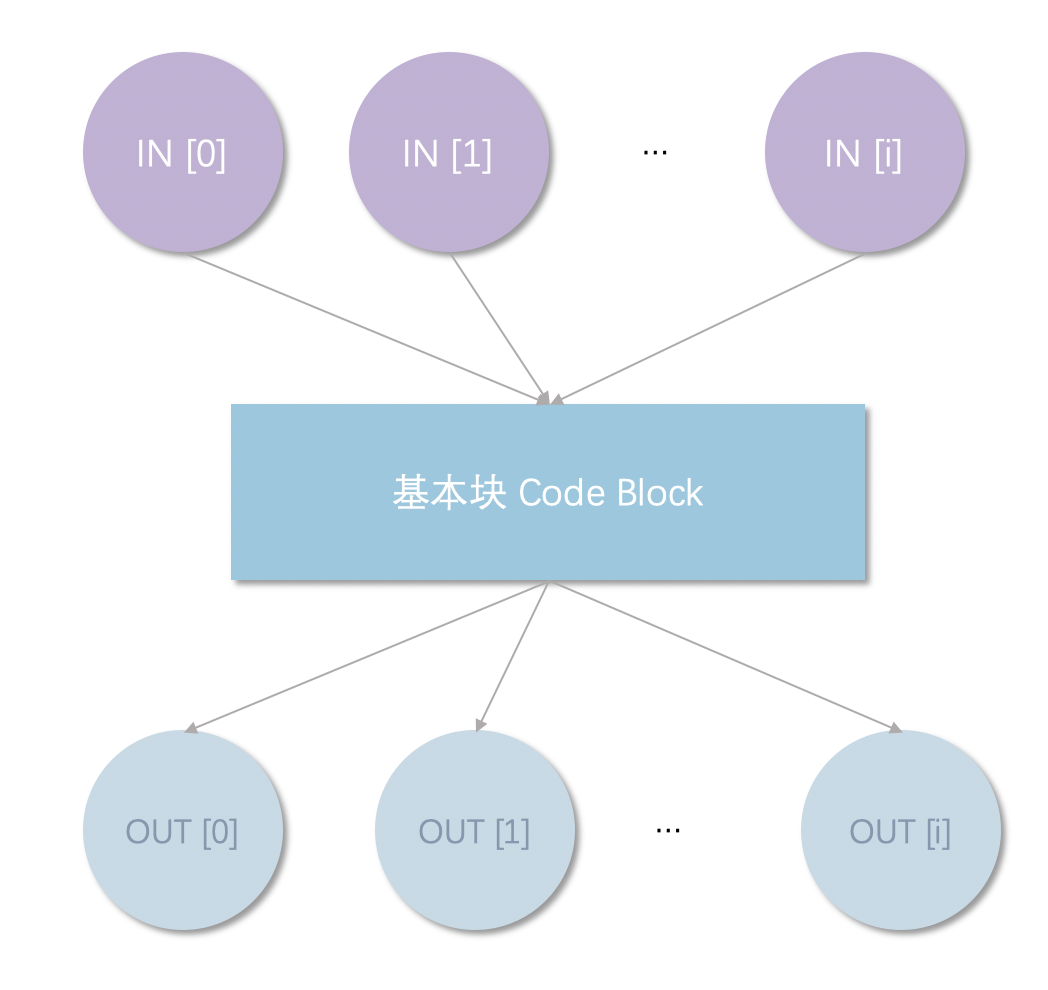$$OUT[B] = f_s(\bigcup_{i}^{0..n}IN[i])$$

$$IN[B] = f_s(\bigcup_{i}^{0..n}OUT[i])$$

$$IN[ENTRY] = \emptyset$$

$$OUT[EXIT] = IN[EXIT]$$

## 到达定值$$\bigcup_{i}^{0..n}IN[i] = IN \cup IN \cup … \cup IN[n]$$

$$f_s(B_i) = (\bigcup_{i}^{0..n}IN[i] - Kill[B_{i}])\cup Gen[B_{i}]$$

Kill Generate
Kill[A] = $\emptyset$; Gen[A] = ${x_{a}, sum_{a}}$
Kill[B] = $\emptyset$; Gen[B] = $\emptyset$
Kill[C] = ${x_{a}, sum_{a}, x_{c}, sum_{c}}$; Gen[C] = ${x_{c}, sum_{c}}$
Kill[D] = $\emptyset$; Gen[D] = $\emptyset$

Block IN OUT
ENTRY $\emptyset$ $\emptyset$
A $\emptyset$ ${x_{a}, sum_{a}}$
B ${x_{a}, sum_{a}, x_{c}, sum_{c}}$ ${x_{a}, sum_{a}, x_{c}, sum_{c}}$
C ${x_{a}, sum_{a}, x_{c}, sum_{c}}$ ${x_{c}, sum_{c}}$
D ${x_{a}, sum_{a}, x_{c}, sum_{c}}$ ${x_{a}, sum_{a}, x_{c}, sum_{c}}$
EXIT ${x_{a}, sum_{a}, x_{c}, sum_{c}}$ ${x_{a}, sum_{a}, x_{c}, sum_{c}}$